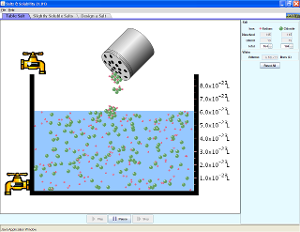Lab 3 - prelab activity - Exploring Concentrations

Lab 3- Solubility and Equilibrium

Help from Luetgens for Moles

Help from Luetgens on equilibrium

Lab 3 - powerpoint

Need help with ionic formulas?  Click here for help and the worksheets done in class.

Simulation.Add different salts to water, then watch them dissolve and achieve a dynamic equilibrium with solid precipitate. Compare the number of ions in solution for highly soluble NaCl to other slightly soluble salts. Relate the charges on ions to the number of ions in the formula of a salt. Calculate Ksp values.

Pracitce writing formulas for ionic compounds.  You must balance the charges on the ions to find the ratio of ions.  Help from Luetgens

Happy Mole Day Oct 23

The concept of moles is introduced to "count" the number of particles.  Ionic charges are used to predict the formulas of ionic compounds.

Lab 3- pre-lab investigation of solutions.  Procedures are designed to measure the concentration (as a variety of ratios of comparison).  Students measure three different volumes (10, 20, and 30 mL) of a single solution of copper (II) sulfate.  After measuring the mass and volume of the solution, the water is removed by boiling and the mass of solute is measured and the mass of water calculated.  Percent by mass, mole fraction, molarity and molality are introduced.

Lab 3.  Saturated solutions are prepared and the concentration of the solution is measured and calculated.  Equilibrium in a saturated solution is described using the equilibrium model introduced in lab 1.  Equilibrium is quantified in the introduction of the equilibrium constant: solubility product, Ksp.  Models ofionic compounds in solution, enthalpy and entropy are applied.